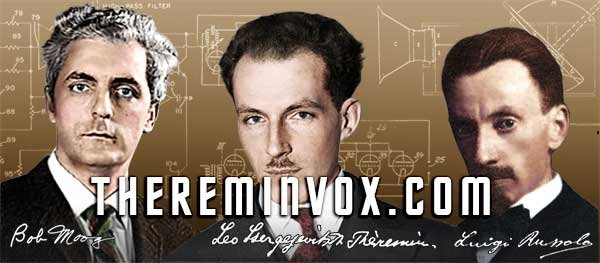PrintMail
 TVOX StoriesFrequency

Frequency is a measurement of the number of cycles repeated per event in a given time. To compute the frequency, one fixes a time interval, counts the number of occurrences of the event, and divides this count by the length of the time interval. The result is presented in units of hertz (Hz) after German physicist Heinrich Rudolf Hertz, where 1 Hz is an event that occurs once per second. Alternatively, one can measure the time between two occurrences of the event (the period) and then compute the frequency as the reciprocal of this time,where T is the period.

## Frequency of Waves

In measuring the frequency of sound, electromagnetic waves (such as radio or light), electrical signals, or other waves, the frequency in hertz is the number of cycles of the repetitive waveform per second.

Frequency has an inverse relationship to the concept of wavelength. The frequency f is equal to the speed v of the wave divided by the wavelength λ of the wave:## Statistical Frequency

In statistics, the frequency of an event is simply the number of times the event occurred in the experiment or the study. These frequencies are often graphically represented in histograms.